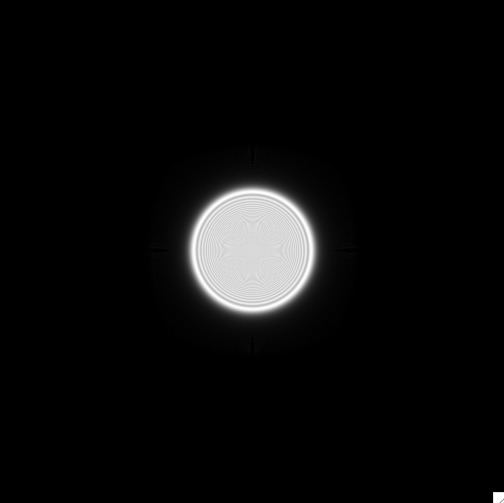# Fresnel number

Updated on
Covid-19The Fresnel number (F), named after the physicist Augustin-Jean Fresnel, is a dimensionless number occurring in optics, in particular in scalar diffraction theory.

For an electromagnetic wave passing through an aperture and hitting a screen, the Fresnel number F is defined as

F = a 2 L λ

where

a is the characteristic size (e.g. radius) of the aperture L is the distance of the screen from the aperture λ is the incident wavelength.

The Fresnel number is a useful concept in physical optics. Conceptually, it is the number of half-period zones in the wavefront amplitude, counted from the center to the edge of the aperture, as seen from the observation point (the center of the imaging screen), where a half-period zone is defined so that the wavefront phase changes by π when moving from one half-period zone to the next. An equivalent definition is that the Fresnel number is the difference, expressed in half-wavelengths, between the slant distance from the observation point to the edge of the aperture and the orthogonal distance from the observation point to the center of the aperture.

The Fresnel number establishes a coarse criterion to define the near and far field approximations. Essentially, if Fresnel number is small - less than roughly 1 - the beam is said to be in the far field . If Fresnel number is larger than 1, the beam is said to be near field . However this criterion does not depend on any actual measurement of the wavefront properties at the observation point.

Another criterion called Gaussian pilot beam allowing to define far and near field conditions, consists to measure the actual wavefront surface curvature for an unaberrated system. In this case the wavefront is planar at the aperture position, when the beam is collimated, or at its focus when the beam is converging/diverging. In detail, within a certain distance from the aperture - the near field - the amount of wavefront curvature is low. Outside this distance - the far field - the amount of wavefront curvature is high. This concept applies equivalently close to the focus.

According to the user manual for the Zemax optical design software, the correct approximation for propagation in the near field follows the angular spectrum method. This approximation works well when at the observation point the distance to the aperture is of the same order as the aperture size. This propagation regime satisfies   F 1 .

The correct approximation for the propagation in the far field is Fresnel diffraction. This approximation works well when at the observation point the distance to the aperture is bigger than the aperture size. This propagation regime verifies   F 1 .

Finally, once at the observation point the distance to the aperture is much bigger than the aperture size, propagation becomes well described by Fraunhofer diffraction. This propagation regime verifies   F 1 .

## The Gaussian pilot beam

This criterion, firstly described by and now adopted in propagation codes like, allows one to determine the realm of application of near and far field approximations taking into account the actual wavefront surface shape at the observation point, to sample its phase without aliasing. This criterion is named Gaussian pilot beam and fixes the best propagation method (among angular spectrum, Fresnel and Fraunhofer diffraction) by looking at the behavior of a Gaussian beam piloted from the aperture position and the observation position.

Near/far field approximations are fixed by the analytical calculation of the Gaussian beam Rayleigh length and by its comparison with the input/output propagation distance. If the ratio between input/output propagation distance and Rayleigh length returns 1 the surface wavefront maintains itself nearly flat along its path, which means that no sampling rescaling is requested for the phase measurement. In this case the beam is said to be near field at the observation point and angular spectrum method is adopted for the propagation. At contrary, once the ratio between input/output propagation distance and Gaussian pilot beam Rayleigh range returns > 1 the surface wavefront gets curvature along the path. In this case a rescaling of the sampling is mandatory for a measurement of the phase preventing aliasing. The beam is said to be far field at the observation point and Fresnel diffraction is adopted for the propagation. Fraunhofer diffraction returns then to be an asymptotic case that applies only when the input/output propagation distance is large enough to consider the quadratic phase term, within the Fresnel diffraction integral, negligible irrespectively to the actual curvature of the wavefront at the observation point.

As the figures explain, the Gaussian pilot beam criterion allows describing the diffractive propagation for all the near/far field approximation cases set by the coarse criterion based on Fresnel number.

## References

Similar Topics
Mike Bath
Lionel Manuel
Dhruv Narayan Singh
Topics

 B i Link H2
 L